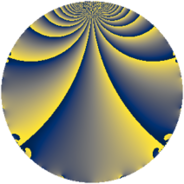# Properties

 Label 575.2.aLevel $575$ Weight $2$ Character orbit 575.a Rep. character $\chi_{575}(1,\cdot)$ Character field $\Q$ Dimension $35$ Newform subspaces $12$ Sturm bound $120$ Trace bound $3$

# Related objects

## Defining parameters

 Level: $$N$$ $$=$$ $$575 = 5^{2} \cdot 23$$ Weight: $$k$$ $$=$$ $$2$$ Character orbit: $$[\chi]$$ $$=$$ 575.a (trivial) Character field: $$\Q$$ Newform subspaces: $$12$$ Sturm bound: $$120$$ Trace bound: $$3$$ Distinguishing $$T_p$$: $$2$$, $$3$$

## Dimensions

The following table gives the dimensions of various subspaces of $$M_{2}(\Gamma_0(575))$$.

Total New Old
Modular forms 66 35 31
Cusp forms 55 35 20
Eisenstein series 11 0 11

The following table gives the dimensions of the cuspidal new subspaces with specified eigenvalues for the Atkin-Lehner operators and the Fricke involution.

$$5$$$$23$$FrickeDim.
$$+$$$$+$$$$+$$$$4$$
$$+$$$$-$$$$-$$$$13$$
$$-$$$$+$$$$-$$$$12$$
$$-$$$$-$$$$+$$$$6$$
Plus space$$+$$$$10$$
Minus space$$-$$$$25$$

## Trace form

 $$35 q + 4 q^{3} + 36 q^{4} + 3 q^{6} + 2 q^{7} - 3 q^{8} + 37 q^{9} + O(q^{10})$$ $$35 q + 4 q^{3} + 36 q^{4} + 3 q^{6} + 2 q^{7} - 3 q^{8} + 37 q^{9} - 2 q^{11} + 17 q^{12} + 4 q^{13} + 22 q^{16} - 4 q^{17} - q^{18} + 20 q^{19} + 6 q^{21} + 10 q^{22} + 3 q^{23} - 2 q^{24} - 13 q^{26} + 4 q^{27} + 14 q^{28} + 4 q^{29} + 12 q^{31} - 6 q^{32} - 10 q^{33} + 30 q^{34} + 3 q^{36} - 4 q^{37} - 30 q^{38} - 16 q^{39} + 8 q^{41} - 8 q^{42} + 12 q^{43} - 16 q^{44} - 2 q^{46} - 16 q^{47} + 49 q^{48} + 55 q^{49} + 10 q^{51} + 35 q^{52} - 10 q^{53} + 9 q^{54} - 18 q^{56} - 43 q^{58} + 4 q^{59} + 34 q^{61} + 19 q^{62} + 8 q^{63} - 23 q^{64} - 78 q^{66} + 2 q^{67} - 28 q^{68} - 4 q^{69} - 56 q^{71} - 51 q^{72} + 4 q^{73} - 44 q^{74} + 50 q^{76} + 4 q^{77} - 11 q^{78} + 20 q^{79} + 67 q^{81} - 5 q^{82} + 38 q^{83} - 82 q^{84} - 16 q^{86} + 8 q^{87} - 22 q^{88} + 10 q^{89} + 70 q^{91} + 6 q^{92} + 42 q^{93} - 49 q^{94} - 61 q^{96} - 28 q^{98} - 36 q^{99} + O(q^{100})$$

## Decomposition of $$S_{2}^{\mathrm{new}}(\Gamma_0(575))$$ into newform subspaces

Label Dim $A$ Field CM Traces A-L signs $q$-expansion
$a_{2}$ $a_{3}$ $a_{5}$ $a_{7}$ 5 23
575.2.a.a $1$ $4.591$ $$\Q$$ None $$-2$$ $$-2$$ $$0$$ $$-1$$ $-$ $-$ $$q-2q^{2}-2q^{3}+2q^{4}+4q^{6}-q^{7}+\cdots$$
575.2.a.b $1$ $4.591$ $$\Q$$ None $$-2$$ $$0$$ $$0$$ $$-1$$ $+$ $+$ $$q-2q^{2}+2q^{4}-q^{7}-3q^{9}+2q^{11}+\cdots$$
575.2.a.c $1$ $4.591$ $$\Q$$ None $$-1$$ $$0$$ $$0$$ $$1$$ $-$ $-$ $$q-q^{2}-q^{4}+q^{7}+3q^{8}-3q^{9}-q^{11}+\cdots$$
575.2.a.d $1$ $4.591$ $$\Q$$ None $$1$$ $$0$$ $$0$$ $$-1$$ $+$ $+$ $$q+q^{2}-q^{4}-q^{7}-3q^{8}-3q^{9}-q^{11}+\cdots$$
575.2.a.e $1$ $4.591$ $$\Q$$ None $$2$$ $$2$$ $$0$$ $$1$$ $-$ $+$ $$q+2q^{2}+2q^{3}+2q^{4}+4q^{6}+q^{7}+\cdots$$
575.2.a.f $2$ $4.591$ $$\Q(\sqrt{5})$$ None $$1$$ $$0$$ $$0$$ $$-2$$ $+$ $+$ $$q+\beta q^{2}+(1-2\beta )q^{3}+(-1+\beta )q^{4}+\cdots$$
575.2.a.g $2$ $4.591$ $$\Q(\sqrt{5})$$ None $$3$$ $$2$$ $$0$$ $$2$$ $+$ $-$ $$q+(1+\beta )q^{2}+q^{3}+3\beta q^{4}+(1+\beta )q^{6}+\cdots$$
575.2.a.h $4$ $4.591$ 4.4.15317.1 None $$-2$$ $$2$$ $$0$$ $$3$$ $+$ $-$ $$q-\beta _{1}q^{2}+(1+\beta _{2})q^{3}+(1+\beta _{1}+\beta _{2}+\cdots)q^{4}+\cdots$$
575.2.a.i $4$ $4.591$ 4.4.5744.1 None $$0$$ $$-2$$ $$0$$ $$-6$$ $-$ $-$ $$q-\beta _{1}q^{2}+(-1+\beta _{1}+\beta _{2})q^{3}+(\beta _{1}+\cdots)q^{4}+\cdots$$
575.2.a.j $4$ $4.591$ 4.4.5744.1 None $$0$$ $$2$$ $$0$$ $$6$$ $-$ $+$ $$q+\beta _{1}q^{2}+(1-\beta _{1}-\beta _{2})q^{3}+(\beta _{1}+\beta _{2}+\cdots)q^{4}+\cdots$$
575.2.a.k $7$ $4.591$ $$\mathbb{Q}[x]/(x^{7} - \cdots)$$ None $$-1$$ $$0$$ $$0$$ $$3$$ $-$ $+$ $$q-\beta _{1}q^{2}+\beta _{5}q^{3}+(2+\beta _{2})q^{4}+(1-\beta _{1}+\cdots)q^{6}+\cdots$$
575.2.a.l $7$ $4.591$ $$\mathbb{Q}[x]/(x^{7} - \cdots)$$ None $$1$$ $$0$$ $$0$$ $$-3$$ $+$ $-$ $$q+\beta _{1}q^{2}-\beta _{5}q^{3}+(2+\beta _{2})q^{4}+(1-\beta _{1}+\cdots)q^{6}+\cdots$$

## Decomposition of $$S_{2}^{\mathrm{old}}(\Gamma_0(575))$$ into lower level spaces

$$S_{2}^{\mathrm{old}}(\Gamma_0(575)) \cong$$ $$S_{2}^{\mathrm{new}}(\Gamma_0(23))$$$$^{\oplus 3}$$$$\oplus$$$$S_{2}^{\mathrm{new}}(\Gamma_0(115))$$$$^{\oplus 2}$$